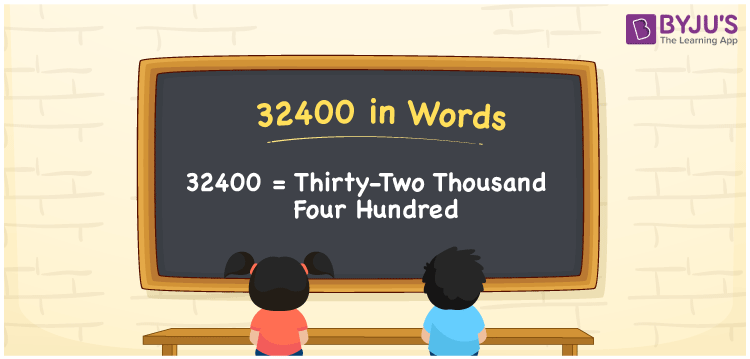# 32400 in Words

We can write 32400 in words is written as Thirty-two thousand four hundred. If you bought a new smart TV that costs Rs. 32400, then you can say, “I bought a new smart TV worth Thirty-two thousand four hundred rupees”. From this, we can say that the word form of the number 32400 is used to define a certain amount or quantity equivalent to 32400. Let’s understand how to convert 32400 into word form with the help of a place value chart.

 32400 in words Thirty-two thousand four hundred Thirty-two thousand four hundred in Numbers 32400

## 32400 in English Words

Generally, we use the English alphabet to write numbers in words. Thus, we can spell 32400 in English words as “Thirty-two thousand four hundred”.## How to Write 32400 in Words?

The number 32400 is a five-digit number, so let’s make a five-column place value chart to convert the numeral 32400 into words.

 Ten thousands Thousands Hundreds Tens Ones 3 2 4 0 0

Here, ones = 0, tens = 0, hundreds = 4, thousands = 2, ten thousands = 3

By expanding these digits based on their place values, we get;

3 × Ten thousand + 2 × Thousand + 4 × Hundred + 0 × Ten + 0 × One

= 3 × 10000 + 2 × 1000 + 4 × 100 + 0 × 10 + 0 × 1

= 30000 + 2000 + 400

= Thirty Thousand + Two thousand + Four hundred

= Thirty-two thousand + Four hundred

= Thirty-two thousand four hundred

As we know, 32400 is a natural number that is the successor of 32399 and the predecessor of 32401.

32400 in words – Thirty-two thousand four hundred

Is 32400 an odd number? – No

Is 32400 an even number? – Yes

Is 32400 a prime number? – No

Is 32400 a composite number? – Yes

Is 32400 a perfect square number? – Yes

What is the square root of 32400? – 180

Is 32400 a perfect cube number? – No

## Frequently Asked Questions on 32400 in Words

Q1

### How do you write 32400 in words?

We can write 32400 in words as given below: 32400 = Thirty-two thousand four hundred
Q2

### How do you write Rs. 32400 in words on a cheque?

On a cheque, we can write Rs. 32400 in words as “Thirty-two thousand four hundred rupees only”.
Q3

### Write the value of 32400/12.

32400/12 = 2700 Therefore, the value of 32400/12 is 2700.